# Chapter 13 Geospatial data in R - Vector

The following activity is available as a template github repository at the following link: https://github.com/VT-Hydroinformatics/12-Intro_Geospatial_Vector

Much of this is adapted from: https://geocompr.robinlovelace.net/index.html Chapter 8

Load necessary packages and data (spData and spDataLarge are data packages)

#install.packages('spDataLarge', repos='https://nowosad.github.io/drat/',
#type='source')

library(tidyverse)
library(sf)
library(raster)
library(dplyr)
library(spData)
library(spDataLarge)
library(tmap)    # for static and interactive maps
library(leaflet) # for interactive maps

theme_set(theme_classic())

## 13.1 Goals

Our goals for this chapter are just to see some of the ways we can wrangle and plot vector spatial data using R. This is by no means the only way and is not an exhaustive demonstration of the packages loaded, but it’ll get us started.

First, we need to define raster and vector spatial data.

Check out the images below for two examples of the same data represented as raster data or vector data.

Vector: Points, lines, polygons, boundaries are crisp regardless of scale Raster: Grid of same sized cells, vales in cells, cell size = resolution (smaller cells, higher resolution)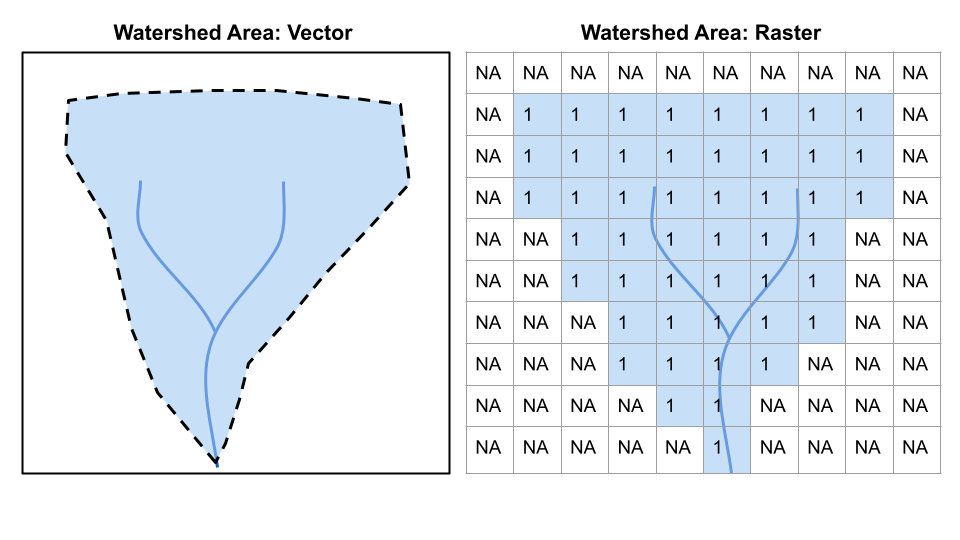Raster vs. Vector 1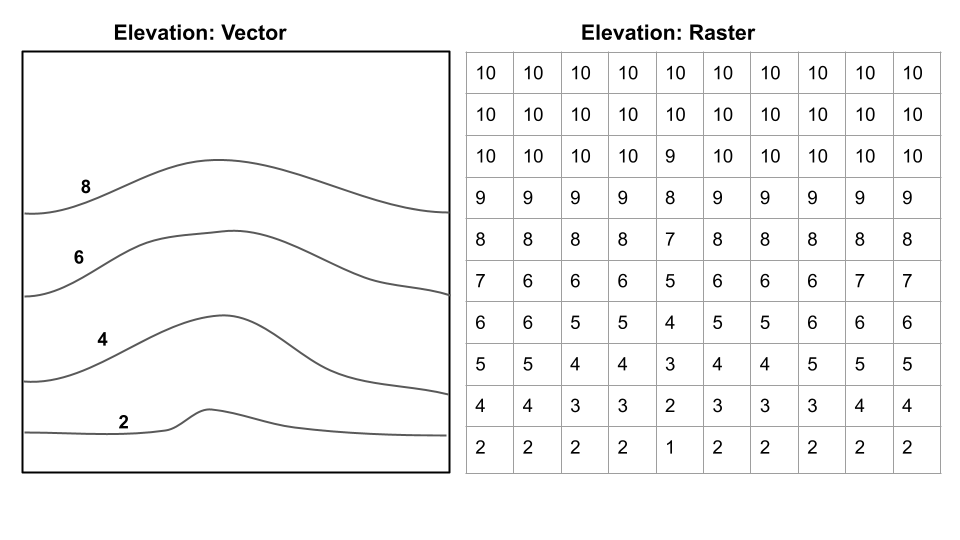Raster vs. Vector 2

Questions from these two images:

What are the advantages/disadvantages of raster/vector for each? Which is best to show on a map for each?  *For elevation, which would be better for calculating slope?

So, today we are sticking to vector data, but then we will be deal primarily with raster elevation data.

## 13.2 Intro to tmap

We are going to mape maps mostly with tmap. But there are several other options.

Let’s look at how tmap works. It uses the same syntax as ggplot: the grammar of graphics.

First we want to set tmap to static map mode. This is what we would want if we were making maps for a manuscript or a paper. You can also make interactive maps with tmap, which we will show later.

Then we will have a look at the us_states data from the data package we loaded above.

What extra information does the data have beyond a regular R object? Play around with it, can you reference columns in the table the same way you would with a regular object?

#mape sure tmap is in static map mode
tmap_mode("plot")
## tmap mode set to plotting
#look at the us_states shapefile data
head(us_states)
## Simple feature collection with 6 features and 6 fields
## Geometry type: MULTIPOLYGON
## Dimension:     XY
## Bounding box:  xmin: -114.8136 ymin: 24.55868 xmax: -71.78699 ymax: 42.04964
## CRS:           EPSG:4269
##   GEOID        NAME   REGION             AREA total_pop_10 total_pop_15
## 1    01     Alabama    South 133709.27 [km^2]      4712651      4830620
## 2    04     Arizona     West 295281.25 [km^2]      6246816      6641928
## 3    08    Colorado     West 269573.06 [km^2]      4887061      5278906
## 4    09 Connecticut Norteast  12976.59 [km^2]      3545837      3593222
## 5    12     Florida    South 151052.01 [km^2]     18511620     19645772
## 6    13     Georgia    South 152725.21 [km^2]      9468815     10006693
##                         geometry
## 1 MULTIPOLYGON (((-88.20006 3...
## 2 MULTIPOLYGON (((-114.7196 3...
## 3 MULTIPOLYGON (((-109.0501 4...
## 4 MULTIPOLYGON (((-73.48731 4...
## 5 MULTIPOLYGON (((-81.81169 2...
## 6 MULTIPOLYGON (((-85.60516 3...

Let’s make a map showing the us_states data. Each state has coordinates to draw it in the dataset, and tmap knows how to deal with that. It uses the same format as ggplot, but instead of ggplot() you will use tm_shape(). Then the geoms are prefixed tm_, so we will use tm_fill to show a map of the US with states filled in with a color.

# Pass the us_states data to tmap and fill the polygons (states)
tm_shape(us_states) +
tm_fill()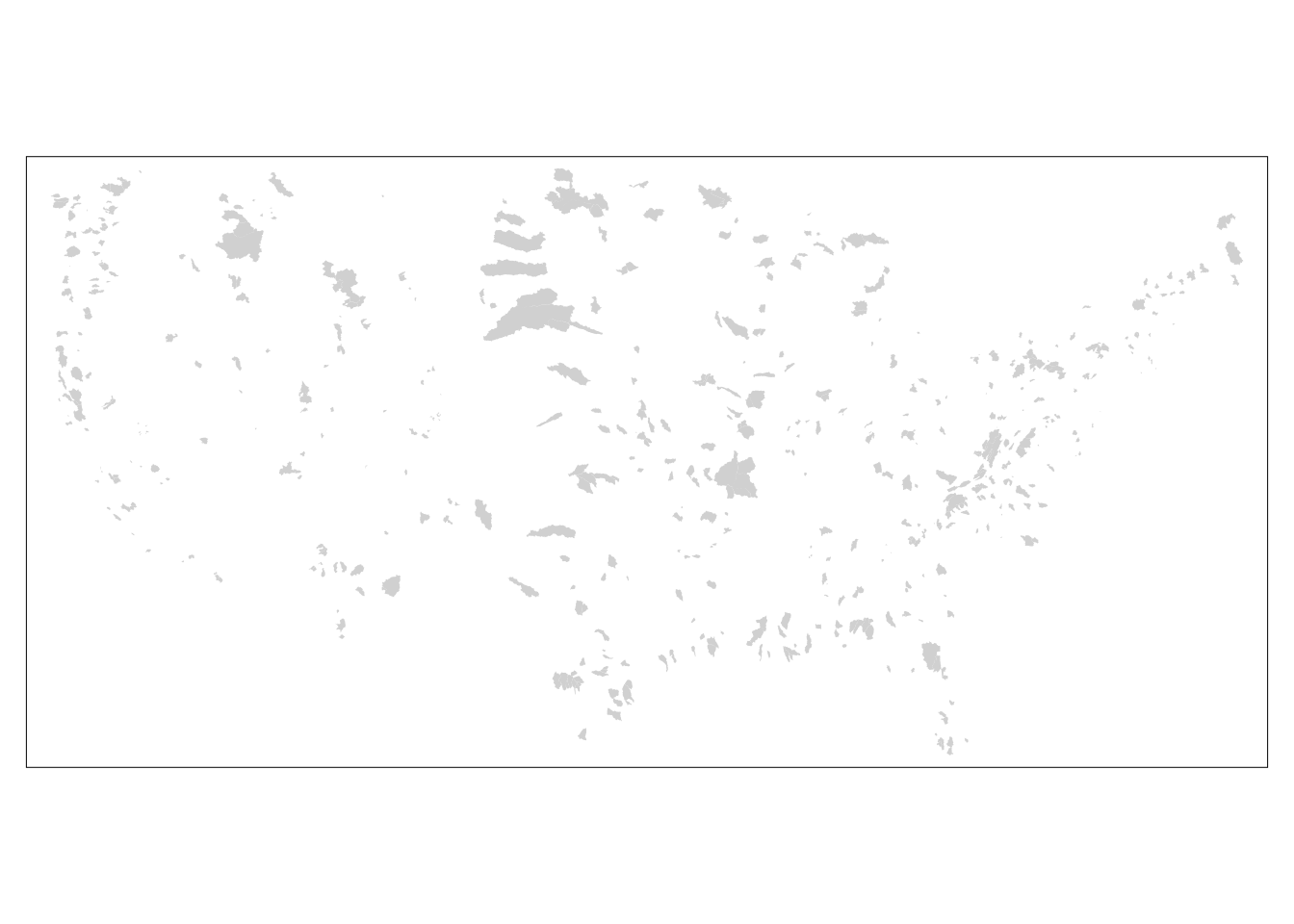If we use tm_borders instead, it will just outline the states.

# Add border layer to shape
tm_shape(us_states) +
tm_borders()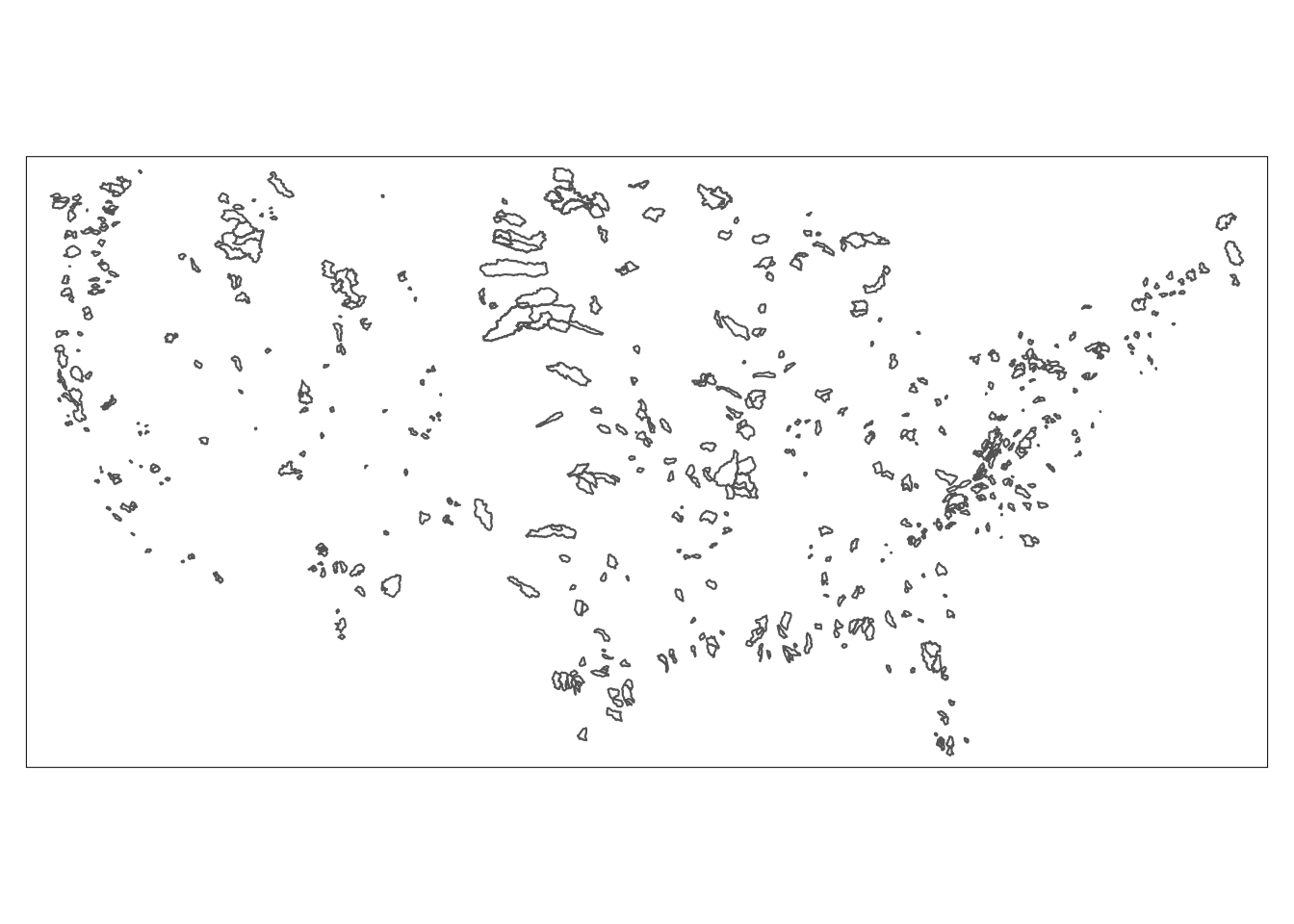Or, like with ggplot, we can add multiple geoms. Let’s do fill AND outline.

# Add fill and border layers to shape
tm_shape(us_states) +
tm_fill() +
tm_borders()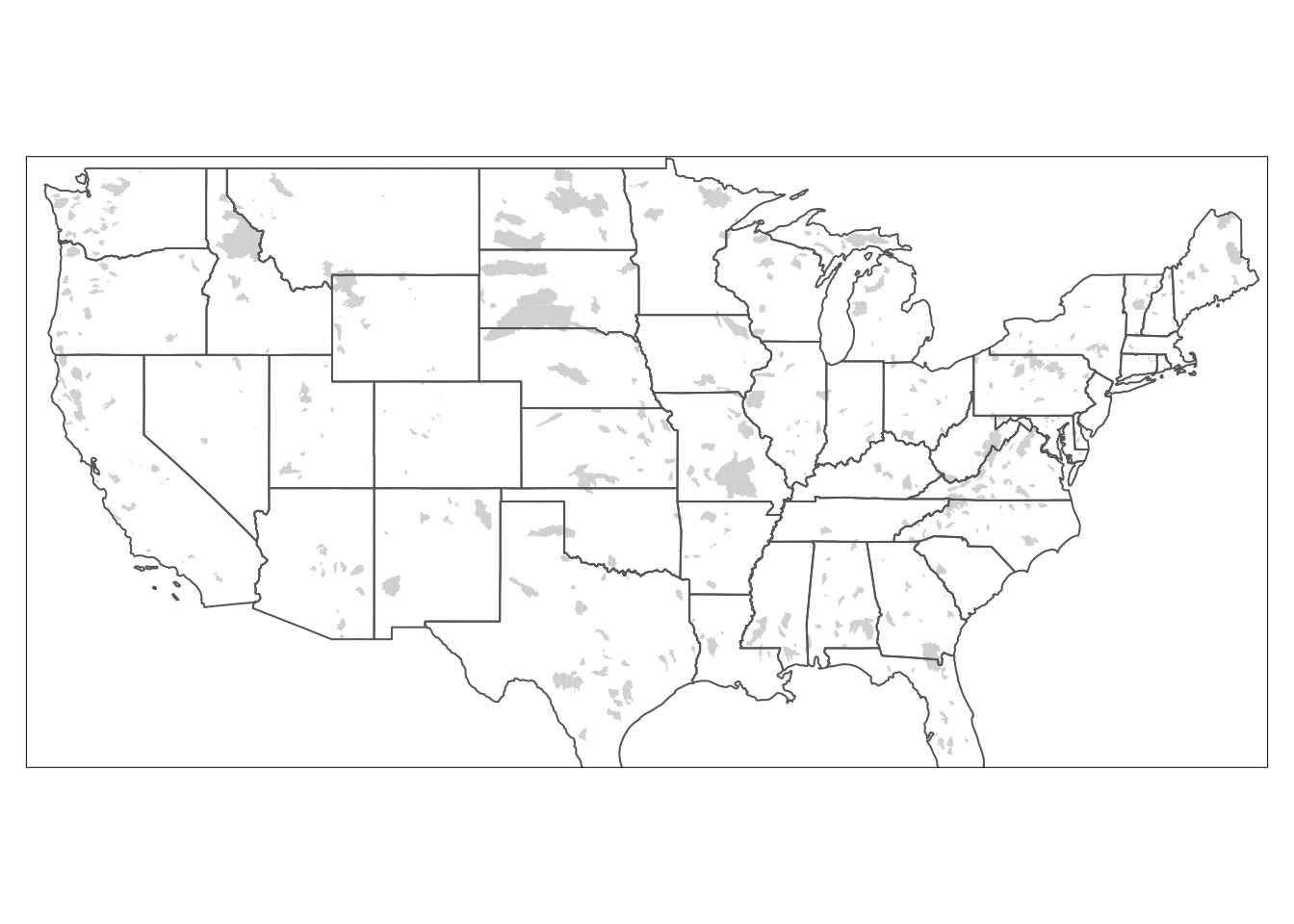We can save these objects to view or edit later just like a ggplot object. We will save the above map as usa.

Then we can use several built in geometries in tmap to add a compass, scale, and title. Note the syntax for specifying the position of the objects. You could do this all in one statement too if you wanted.

#Save basic map object as "usa"
usa <- tm_shape(us_states) +
tm_fill() +
tm_borders()

usa +
tm_compass(type = "8star", position = c("right", "bottom")) +
tm_scale_bar(position = c("left", "bottom"))+
tm_layout(title = "United States", title.position = c("right", "TOP"))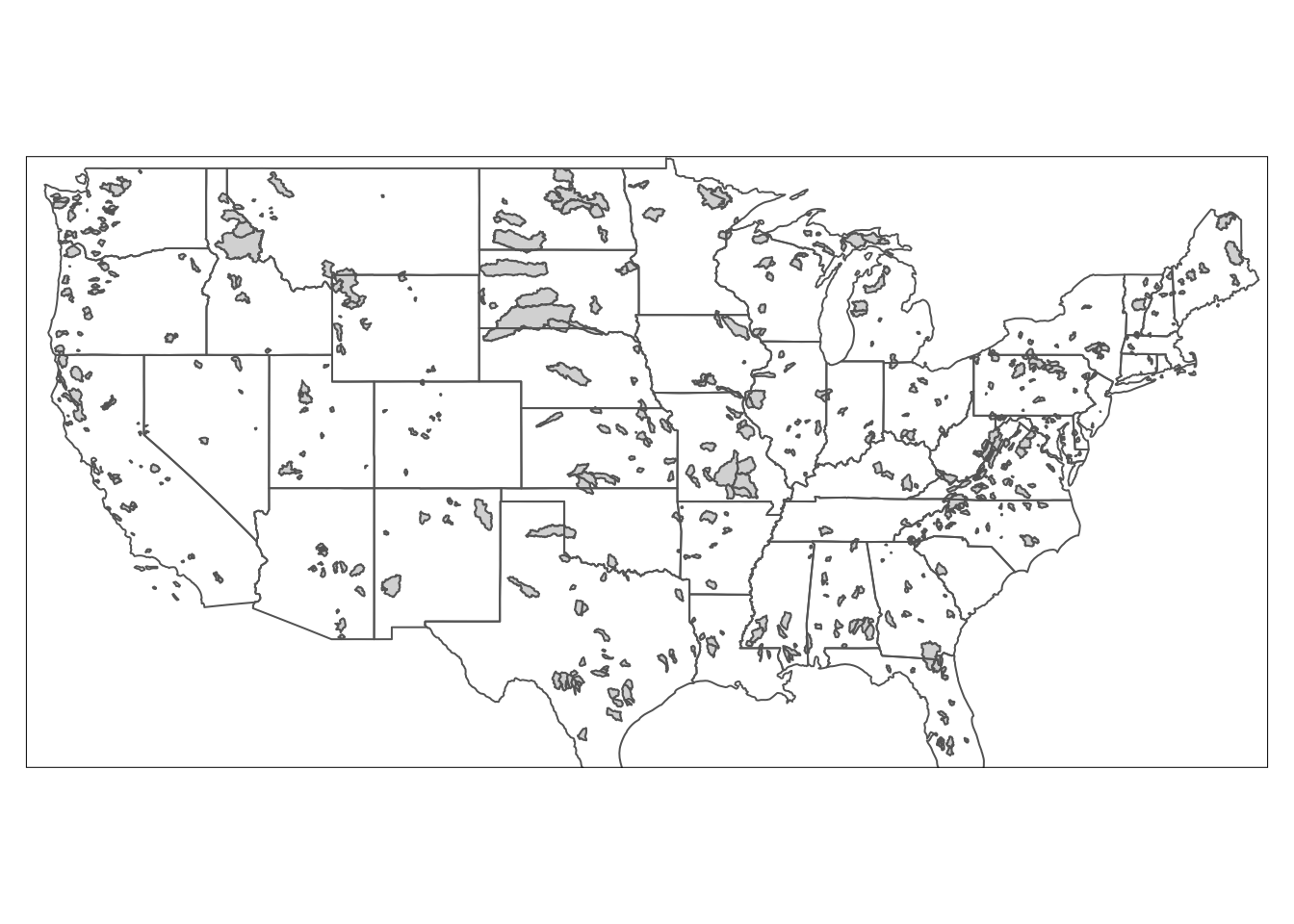Below is an example of how to edit the “symbology” of the map. In other words, we want to color each of the polygons depending on a variable. Here we make the states more blue if they have a higher population.

The syntax below is basically (pseudo code):

Represent us_states as shapes +
color the shapes based on total_pop_10, use 10 colors, use the Blues palette
add a legend in the bottom right, add some space for the title, define the title, position the title
add a compass at the bottom left
add a scale bar at the bottom left

tm_shape(us_states) +
tm_polygons(col = "total_pop_10", n = 10, palette = "Blues")+
tm_layout(legend.position = c("right", "bottom"),
inner.margins = 0.1,
title = "United States Population in 2010",
title.position = c("center", "TOP"))+
tm_compass(type = "8star", position = c("left", "bottom")) +
tm_scale_bar(position = c("left", "bottom"))
## Scale bar set for latitude km and will be different at the top and bottom of the map.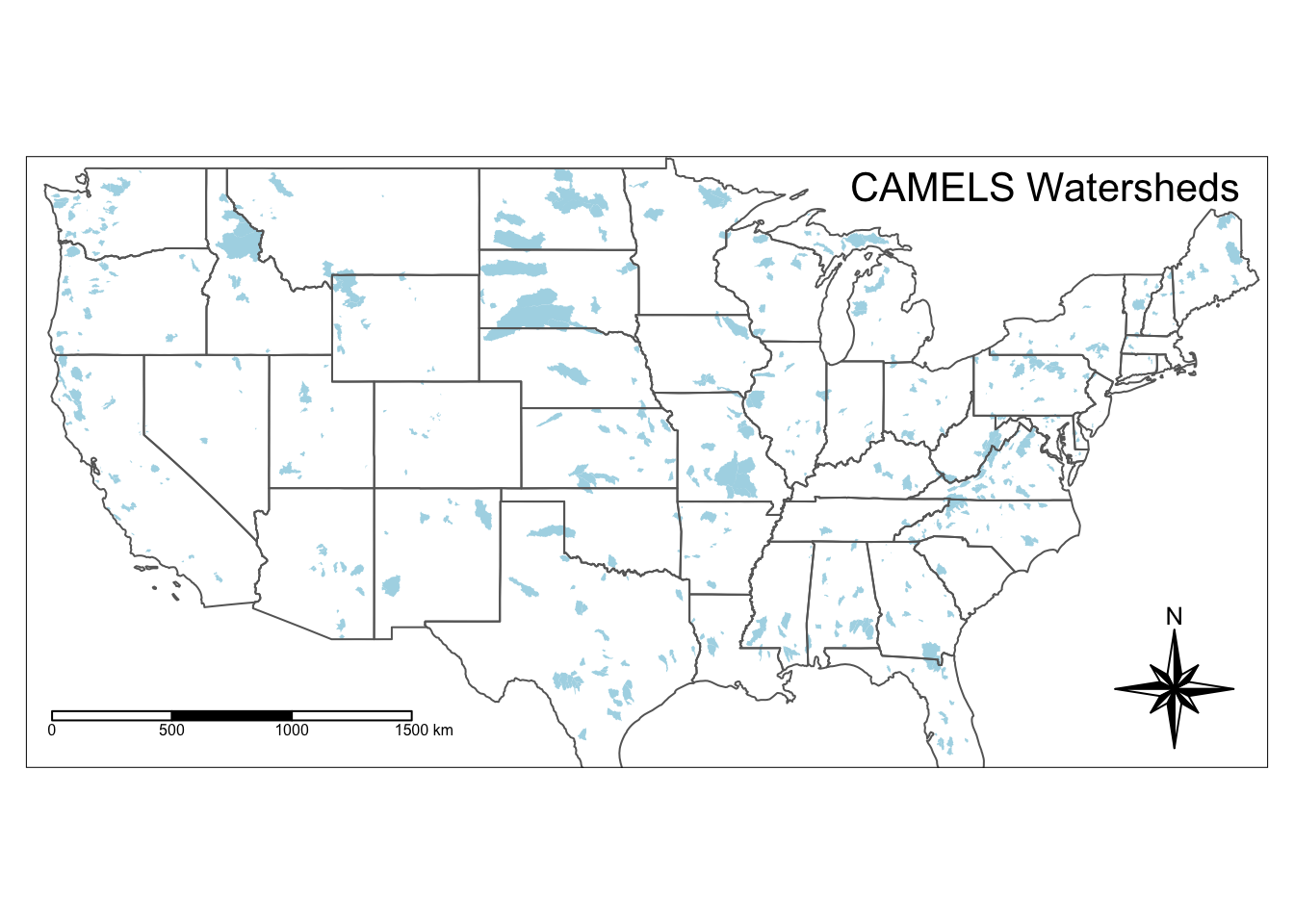## 13.3 Data wrangling with tidyverse principles

You can use the same techniques as with other data to change or filter the spatial data. Below we filter to show just Virginia and then just to show the southern region. Note when we looked at us_states above there is a column called NAME for the state names and REGION for the region name.

us_states %>% filter(NAME == "Virginia") %>%
tm_shape() +
tm_fill() +
tm_borders()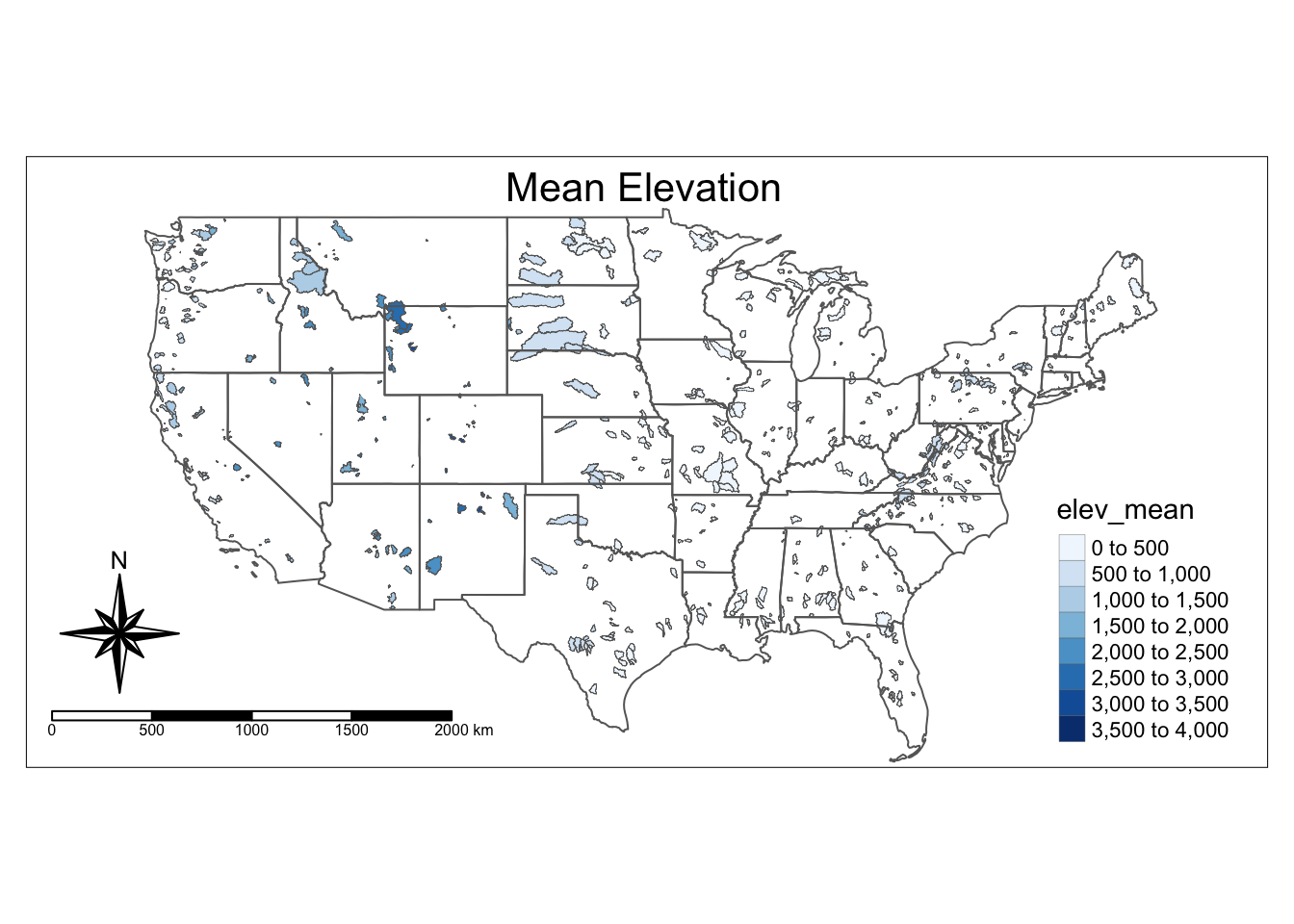Here we filter to the southern region and also add text labels with tm_text.

us_states %>% filter(REGION == "South") %>%
tm_shape() +
tm_fill() +
tm_borders() +
tm_text("NAME", size = 0.7)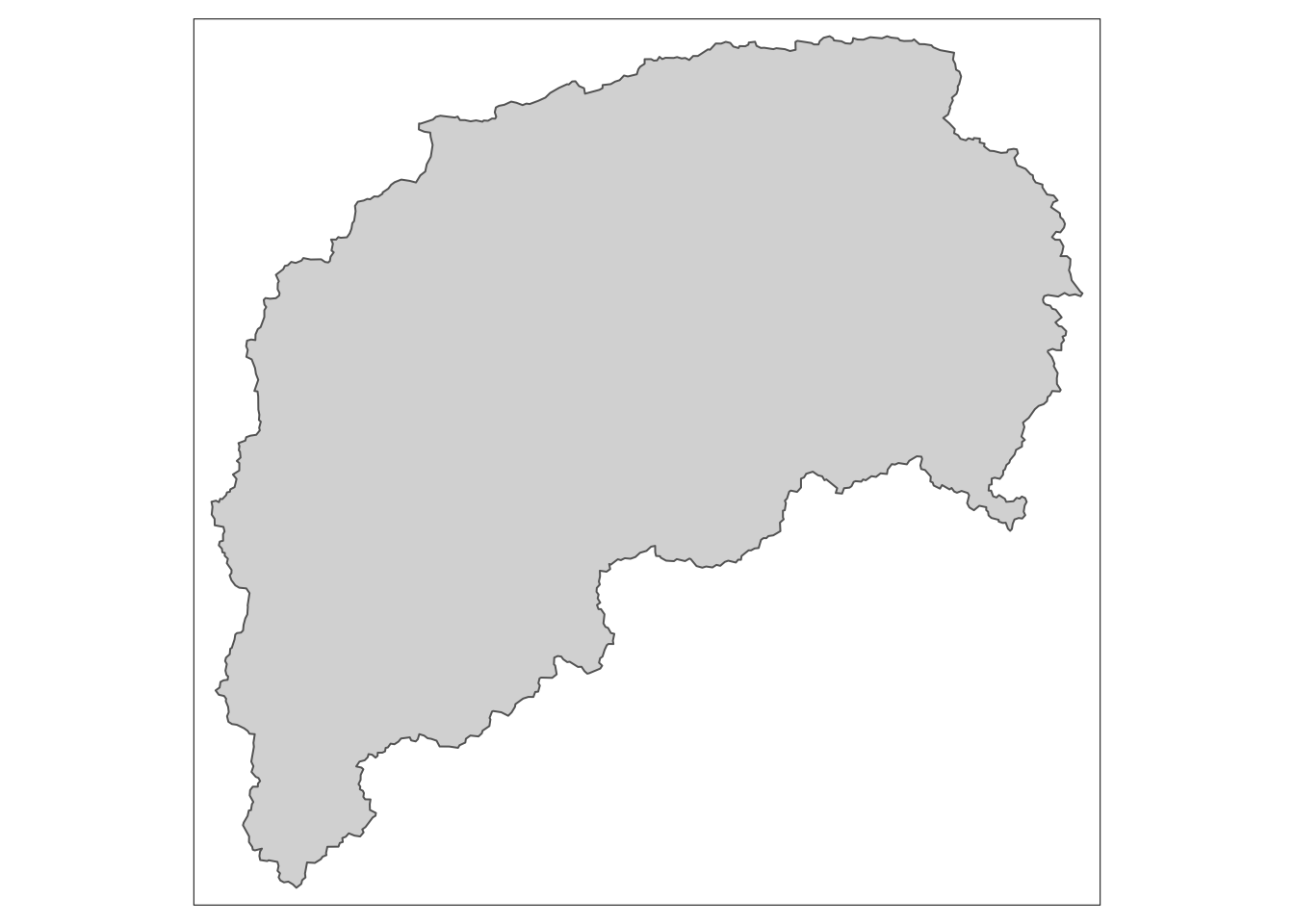In addition to filtering, we can use the data in the geospatial table to calculate additional parameters, just like with a normal object. Below we calculate growth by subtracting the 2010 population from the 2015 population, and percent growth but dividing 2015-2010 by population in 2010 and multiplying by 100.

us_states <- us_states %>%
mutate(growth = total_pop_15 - total_pop_10) %>%
mutate(growthPer = ((total_pop_15-total_pop_10)/total_pop_10) * 100)

head(us_states)
## Simple feature collection with 6 features and 8 fields
## Geometry type: MULTIPOLYGON
## Dimension:     XY
## Bounding box:  xmin: -114.8136 ymin: 24.55868 xmax: -71.78699 ymax: 42.04964
## CRS:           EPSG:4269
##   GEOID        NAME   REGION             AREA total_pop_10 total_pop_15
## 1    01     Alabama    South 133709.27 [km^2]      4712651      4830620
## 2    04     Arizona     West 295281.25 [km^2]      6246816      6641928
## 3    08    Colorado     West 269573.06 [km^2]      4887061      5278906
## 4    09 Connecticut Norteast  12976.59 [km^2]      3545837      3593222
## 5    12     Florida    South 151052.01 [km^2]     18511620     19645772
## 6    13     Georgia    South 152725.21 [km^2]      9468815     10006693
##                         geometry  growth growthPer
## 1 MULTIPOLYGON (((-88.20006 3...  117969  2.503241
## 2 MULTIPOLYGON (((-114.7196 3...  395112  6.325014
## 3 MULTIPOLYGON (((-109.0501 4...  391845  8.018009
## 4 MULTIPOLYGON (((-73.48731 4...   47385  1.336356
## 5 MULTIPOLYGON (((-81.81169 2... 1134152  6.126703
## 6 MULTIPOLYGON (((-85.60516 3...  537878  5.680521

Now we can plot our newly calculated data by controlling color with that new column name.

tm_shape(us_states) +
tm_polygons(col = "growthPer", n = 7,
palette = "Blues", title = "% of 2010 Pop")+ #do growth and growth per
tm_layout(legend.position = c("right", "bottom"),
inner.margins = 0.1,
title = "United States Population Growth 2010-2015",
title.position = c("center", "TOP"))+
tm_compass(type = "8star", position = c("left", "bottom")) +
tm_scale_bar(position = c("left", "bottom"))
## Scale bar set for latitude km and will be different at the top and bottom of the map.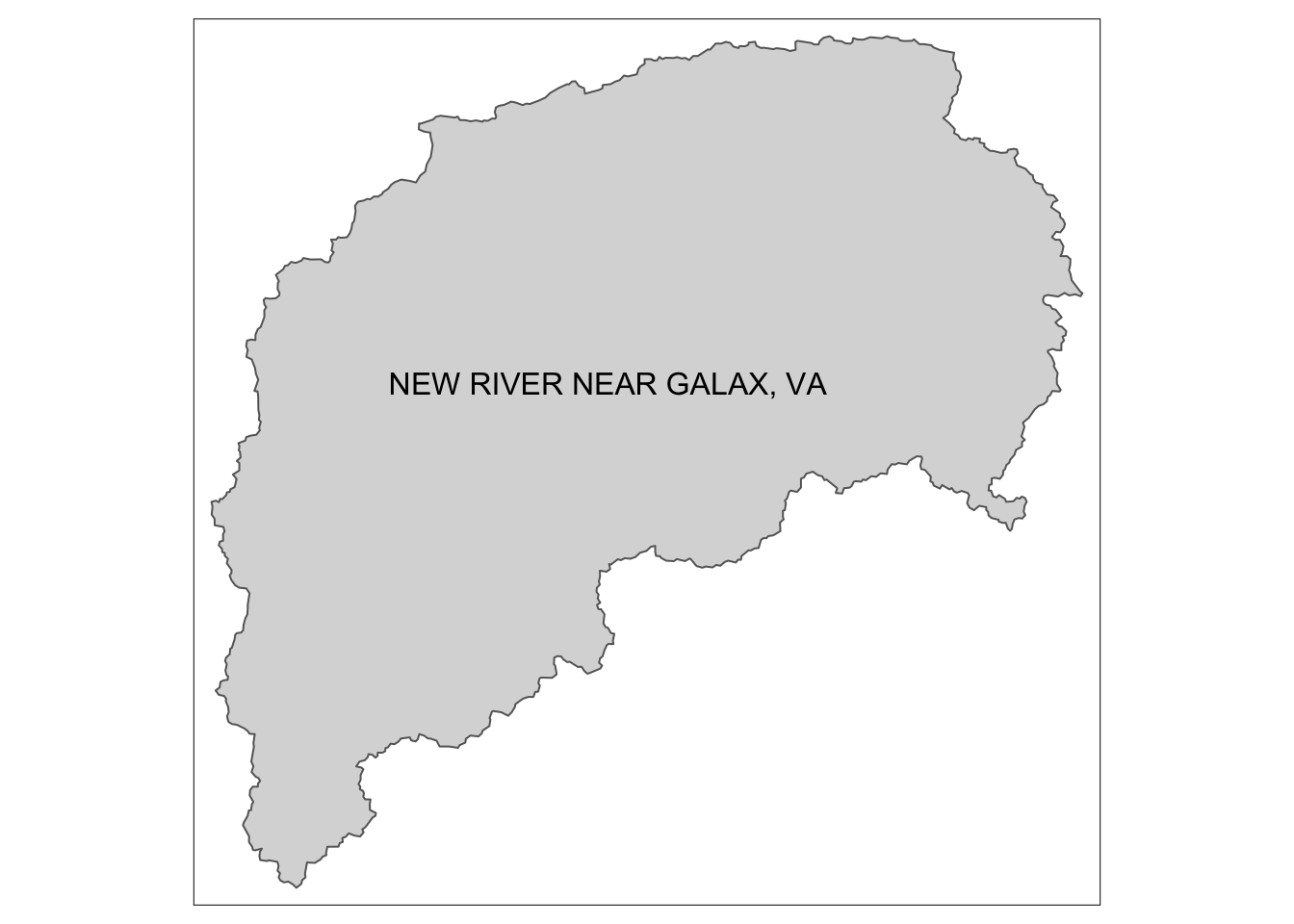## 13.4 Add non-spatial data to spatial data with a join

We have been using us_states. There is another dataset called us_states_df that has even more data, but it is just a regular tibble, not a geospatial file.

We need to attach data from us_states_df to the us_states geospatial file based on state name. How in the world will we do that?

A JOIN!!

In us_states the state names are in a column called NAME and in us_states_df they are in a column called state. So when we do the join, we need to tell R that these columns are the same and we want to use them to match the values. We will do a left join to accomplish this.

To review joins, check out chapter 7

#this says: join us_states and us_states_df by using the NAME and state columns
st_income <- left_join(us_states, us_states_df, by = c("NAME" = "state"))

head(st_income)
## Simple feature collection with 6 features and 12 fields
## Geometry type: MULTIPOLYGON
## Dimension:     XY
## Bounding box:  xmin: -114.8136 ymin: 24.55868 xmax: -71.78699 ymax: 42.04964
## CRS:           EPSG:4269
##   GEOID        NAME   REGION             AREA total_pop_10 total_pop_15  growth
## 1    01     Alabama    South 133709.27 [km^2]      4712651      4830620  117969
## 2    04     Arizona     West 295281.25 [km^2]      6246816      6641928  395112
## 3    08    Colorado     West 269573.06 [km^2]      4887061      5278906  391845
## 4    09 Connecticut Norteast  12976.59 [km^2]      3545837      3593222   47385
## 5    12     Florida    South 151052.01 [km^2]     18511620     19645772 1134152
## 6    13     Georgia    South 152725.21 [km^2]      9468815     10006693  537878
##   growthPer median_income_10 median_income_15 poverty_level_10 poverty_level_15
## 1  2.503241            21746            22890           786544           887260
## 2  6.325014            26412            26156           933113          1180690
## 3  8.018009            29365            30752           584184           653969
## 4  1.336356            32258            33226           314306           366351
## 5  6.126703            24812            24654          2502365          3180109
## 6  5.680521            25596            25588          1445752          1788947
##                         geometry
## 1 MULTIPOLYGON (((-88.20006 3...
## 2 MULTIPOLYGON (((-114.7196 3...
## 3 MULTIPOLYGON (((-109.0501 4...
## 4 MULTIPOLYGON (((-73.48731 4...
## 5 MULTIPOLYGON (((-81.81169 2...
## 6 MULTIPOLYGON (((-85.60516 3...

And now we can plot this formerly non-spatial data on our map.

#now the us_states_df columns are available for us to use when mapping
tm_shape(st_income) +
tm_polygons(col = "median_income_10", n = 7)+
tm_compass(type = "8star", position = c("right", "bottom")) +
tm_scale_bar(position = c("right", "bottom"))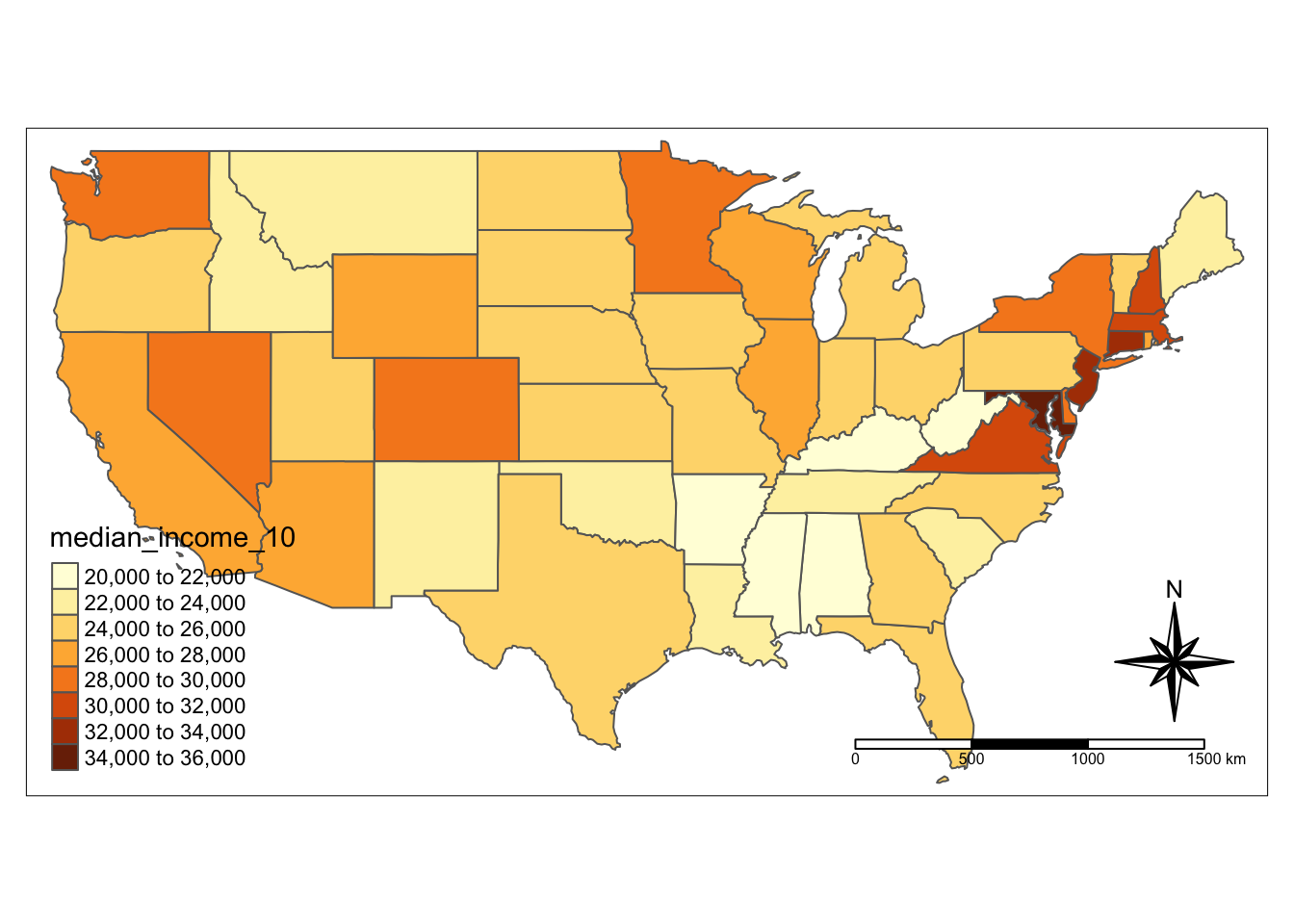## 13.5 Plot maps side by side

Just like we can use facets in ggplot, we can use facets to show multiple maps. Below we show median income in 2010 and 2015 next to each other using tm_facets.

facets = c("median_income_10", "median_income_15")

tm_shape(st_income) +
tm_polygons(facets) +
tm_facets(ncol = 1, sync = TRUE)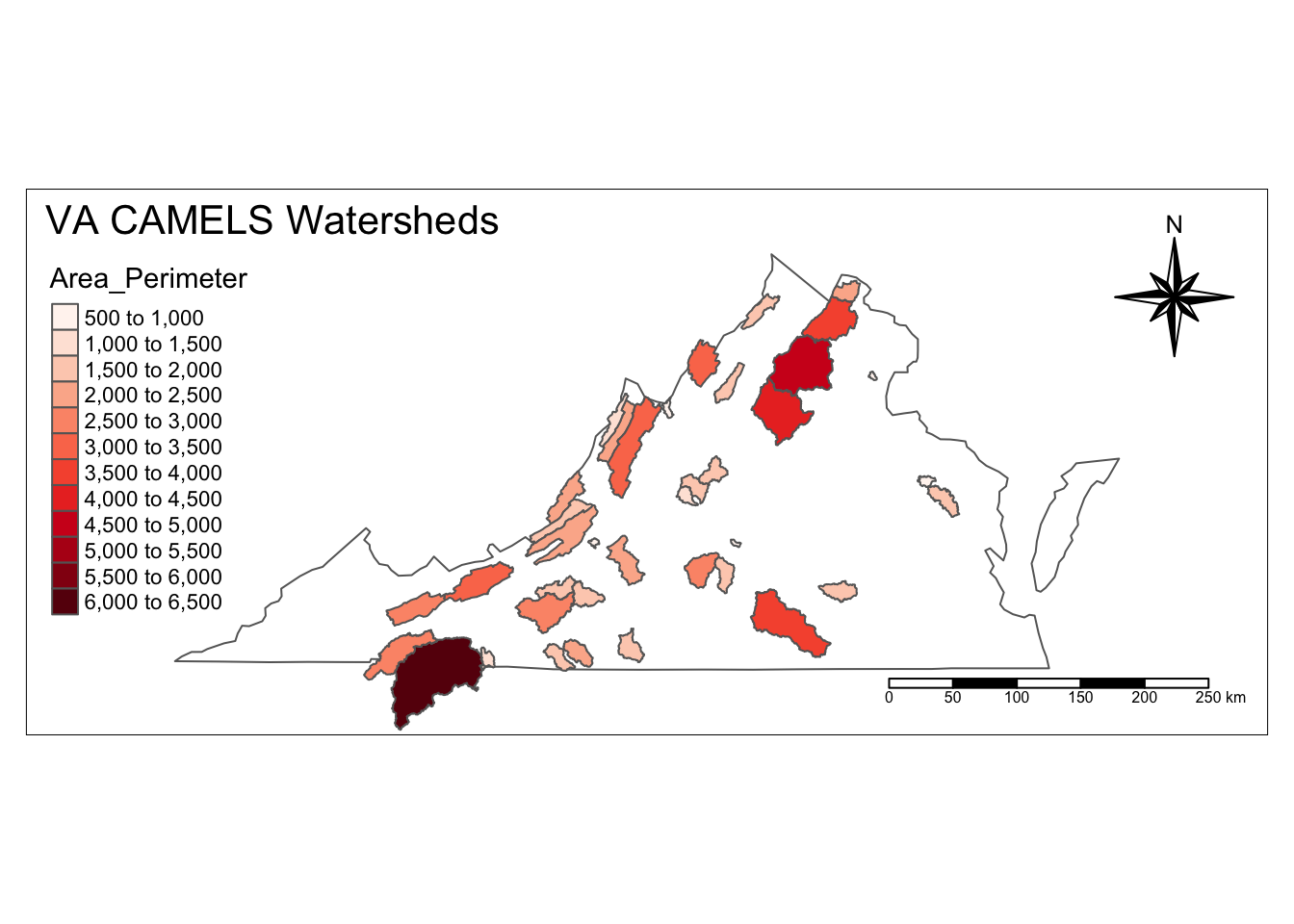## 13.6 Built in styles, like themes in ggplot

We can use these styles with tm_style. Try “classic,” “coblat,” or “col_blind” below.

tm_shape(us_states)+
tm_polygons(col = "growthPer", n = 7,
palette = "Blues", title = "% of 2010 Pop")+ #do growth and growth per
tm_style("classic") #try cobalt, bw, col_blind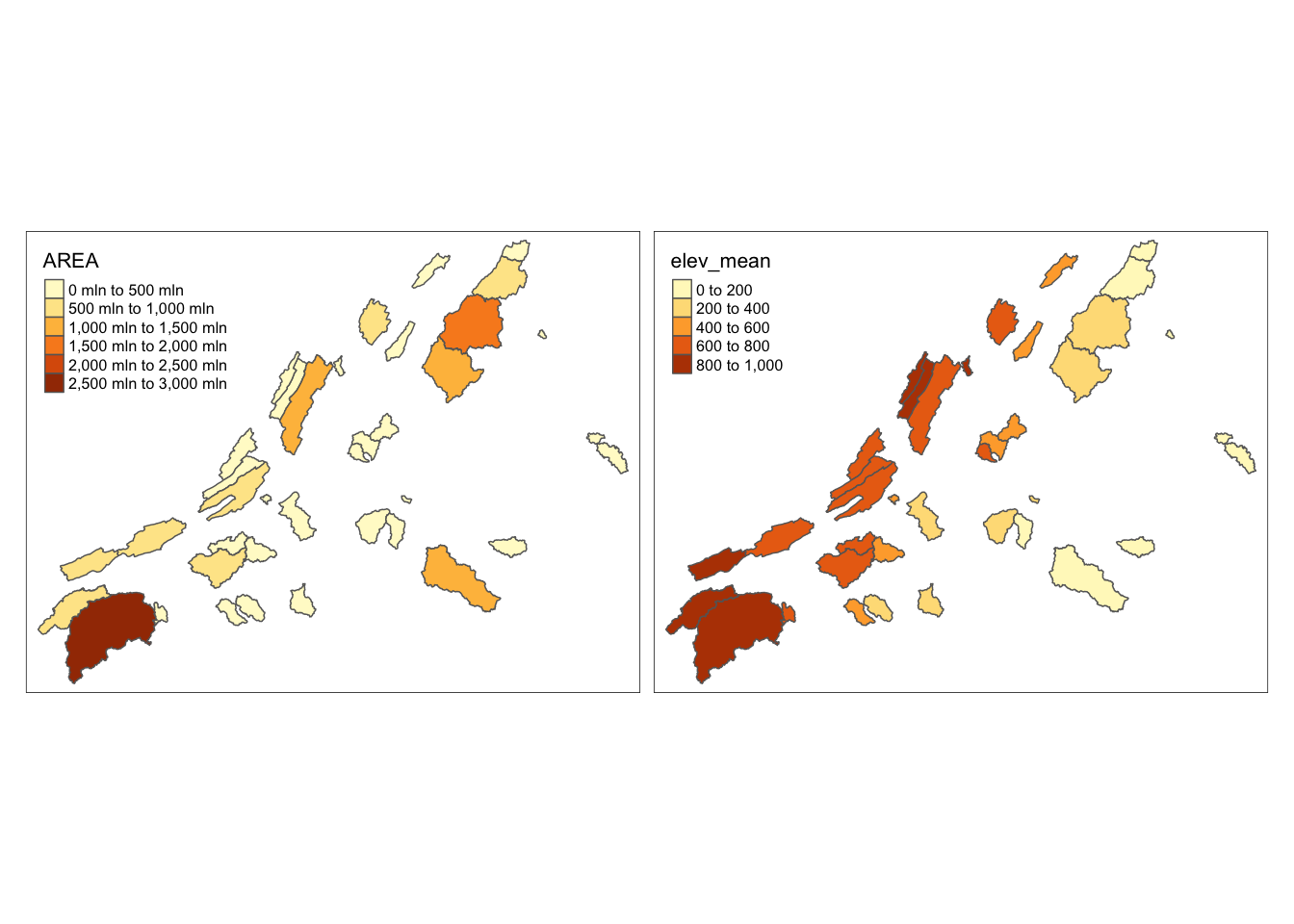## 13.7 Interactive Maps

### 13.7.1 tmap

You can also generate maps that you can interact with, as opposed to static maps, that we have been using before. If you are generating a map for an app or webpage, this may be a good choice. But for a pdf report, the static maps are more appropriate.

In tmap all you have to do is run tmap_mode(“view”) and it will create an interactive map with the exact same syntax! To switch back to a static map, run tmap_mode(“plot”)

Also in this chunk we see how to add a basemap to a tmap object.

tmap_mode("view")
## tmap mode set to interactive viewing
popgrowth <- tm_shape(us_states) +
tm_polygons(col = "growthPer", n = 7,
palette = "Blues", title = "% of 2010 Pop",
alpha = 0.7)+ #mess with alpha
tm_layout(legend.position = c("right", "bottom"),
inner.margins = 0.1,
title = "United States Population Growth 2010-2015",
title.position = c("center", "TOP"))+
tm_compass(type = "8star", position = c("left", "bottom")) +
tm_scale_bar(position = c("left", "bottom"))

popgrowth + tm_basemap(server = "OpenTopoMap")
## Compass not supported in view mode.
## legend.postion is used for plot mode. Use view.legend.position in tm_view to set the legend position in view mode.

### 13.7.2 Leaflet

Leaflet is another way to make interactive maps. It’s syntax is very different, as you can see below. But depending on what functionality you need, it could be a better choice.

leaflet(us_states) %>%
fillColor = ~colorQuantile("YlOrRd", total_pop_10)(total_pop_10))
## Warning: sf layer has inconsistent datum (+proj=longlat +ellps=GRS80 +towgs84=0,0,0,0,0,0,0 +no_defs ).
## Need '+proj=longlat +datum=WGS84'